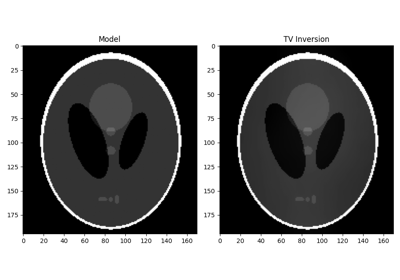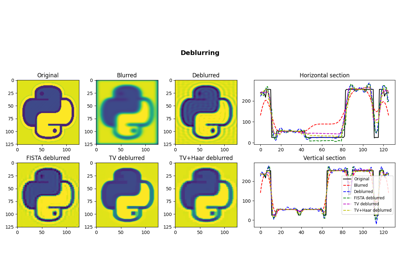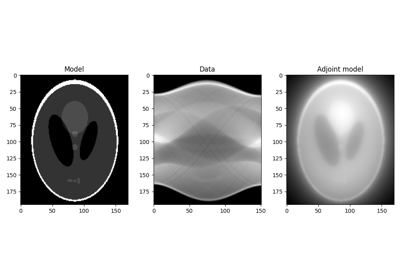# pylops.optimization.sparsity.splitbregman#

pylops.optimization.sparsity.splitbregman(Op, y, RegsL1, x0=None, niter_outer=3, niter_inner=5, RegsL2=None, dataregsL2=None, mu=1.0, epsRL1s=None, epsRL2s=None, tol=1e-10, tau=1.0, restart=False, show=False, itershow=(10, 10, 10), show_inner=False, callback=None, **kwargs_lsqr)[source]#

Split Bregman for mixed L2-L1 norms.

Solve an unconstrained system of equations with mixed $$L_2$$ and $$L_1$$ regularization terms given the operator Op, a list of $$L_1$$ regularization terms RegsL1, and an optional list of $$L_2$$ regularization terms RegsL2.

Parameters
Oppylops.LinearOperator

Operator to invert

ynumpy.ndarray

Data

RegsL1list

$$L_1$$ regularization operators

x0numpy.ndarray, optional

Initial guess

niter_outerint

Number of iterations of outer loop

niter_innerint

Number of iterations of inner loop of first step of the Split Bregman algorithm. A small number of iterations is generally sufficient and for many applications optimal efficiency is obtained when only one iteration is performed.

RegsL2list

Additional $$L_2$$ regularization operators (if None, $$L_2$$ regularization is not added to the problem)

dataregsL2list, optional

$$L_2$$ Regularization data (must have the same number of elements of RegsL2 or equal to None to use a zero data for every regularization operator in RegsL2)

mufloat, optional

Data term damping

epsRL1slist

$$L_1$$ Regularization dampings (must have the same number of elements as RegsL1)

epsRL2slist

$$L_2$$ Regularization dampings (must have the same number of elements as RegsL2)

tolfloat, optional

Tolerance. Stop outer iterations if difference between inverted model at subsequent iterations is smaller than tol

taufloat, optional

Scaling factor in the Bregman update (must be close to 1)

restartbool, optional

The unconstrained inverse problem in inner loop is initialized with the initial guess (True) or with the last estimate (False)

showbool, optional

Display iterations log

itershowtuple, optional

Display set log for the first N1 steps, last N2 steps, and every N3 steps in between where N1, N2, N3 are the three element of the list.

show_innerbool, optional

Display inner iteration logs of lsqr

callbackcallable, optional

Function with signature (callback(x)) to call after each iteration where x is the current model vector

**kwargs_lsqr

Arbitrary keyword arguments for scipy.sparse.linalg.lsqr solver used to solve the first subproblem in the first step of the Split Bregman algorithm.

Returns
xinvnumpy.ndarray

Inverted model

itn_outint

Iteration number of outer loop upon termination

costnumpy.ndarray, optional

History of cost function through iterations

Notes

## Examples using pylops.optimization.sparsity.splitbregman#Total Variation (TV) Regularization

Total Variation (TV) Regularization05. Image deblurring

05. Image deblurring16. CT Scan Imaging

16. CT Scan Imaging